# Bihar Board Class 10th Maths Solutions Chapter 6 Triangles Ex 6.4

Bihar Board Class 10th Maths Solutions Chapter 6 Triangles Ex 6.4 Textbook Questions and Answers.

## BSEB Bihar Board Class 10th Maths Solutions Chapter 6 Triangles Ex 6.4Question 1.
Let ∆ ABC ~ ∆ DEF and their areas be, respectively, 64 cm² and 121 cm². If EF = 15.4 cm, find BC.
Solution: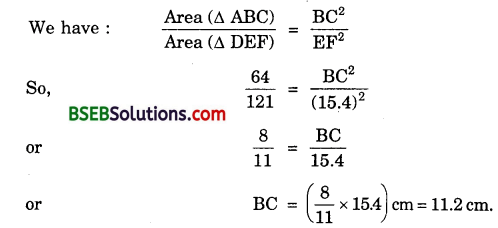Question 2.
Diagonals of a trapezium ABCD with AB || DC intersect each other at the point O. If AB = 2 CD, find the ratio of the areas of triangles AOB and COD.
Solution:
In ∆s AOB and COD, we have :
∠AOB = ∠COD [Vert.opp.∠s]
and ∠OAB = ∠OCD [Alternate ∠s]
∴ By AA criterion of similarity, we have: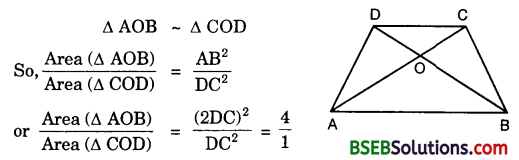Hence, area (∆ AOB) : area (∆ COD) = 4 : 1Question 3.
In the figure, ABC and DBC are two triangles on the same base BC. If AD intersects BC at O, show that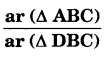= $$\frac { AO }{ DO }$$.Solution:
Given : Two As ABC and DBC which stand on the same base BC but on the opposite sides of BC.
To prove :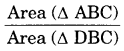= $$\frac { AO }{ DO }$$.
Construction : Draw AE ⊥ BC and DF ⊥ BC.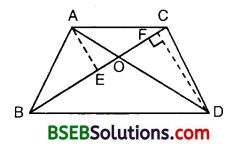Proof:
In ∆s AOE and DOF, we have :
∠AEO = ∠DFO = 90°
∠AOE = ∠DOF [Vertically opp. ∠s]
∴ By AA criterion of similarity, we have :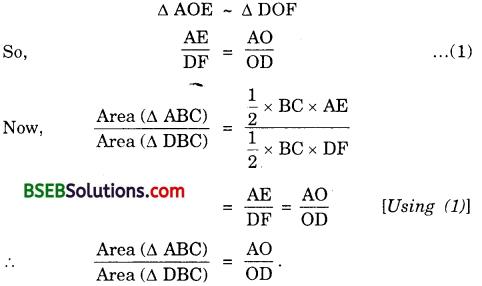Question 4.
If the areas of two similar triangles are equal, prove that they are congruent.
Solution:
Given : Two ∆s ABC and DEF such that ∆ ABC – ∆ DEF
and Area (∆ ABC) = Area (∆ DEF)
To prove : ∆ ABC ≅ ∆ DEF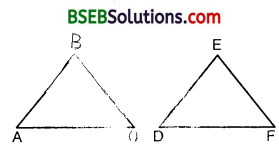Proof:
∆ ABC ~ ∆ DEF
So, ∠A = ∠D, ∠B = ∠E, ∠C = ∠F
and $$\frac { AB }{ DE }$$ = $$\frac { BC }{ EF }$$ = $$\frac { AC }{ DF }$$
To establish ∆ ABC ≅ ∆ DEF, it is sufficient to prove that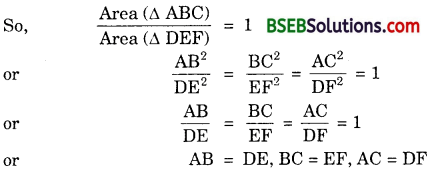Hence ∆ ABC ≅ ∆ DEF [By SSS]Question 5.
D, E and F are respectively the mid-points of sides AB, BC and CA of ∆ ALBC. Find the ratio of the areas of ∆ DEF and ∆ ABC.
Solution:
Since D and E are respectively, the mid-points of the sides AB and BC of ∆ ABC, therefore
DE [| AC, or DE || FC … (1)
Since D and F are respectively the mid-points of the sides AB and AC
∆ ABC, thereforeDF || BC, or DF || EC … (2)
From (1) and (2), we can say that DECF is a parallelogram.
Now, in ∆s DEF and ABC, we have :
∠DEF = ∠A [Opp. Zs of ||gm ADEF]
and ∠EDF = ∠C [Opp. Zs of ||gm DECF]
∴ By AA criterion of similarity, we have :
∆ DE ≅ ∆ ACHence, Area (∆ DEF) : Area (∆ ABC) =1:4.

Question 6.
Prove that the ratio of the areas of two similar triangles is equal to the square of the ratio of their corresponding medians.
Solution:
Given : ∆ ABC ~ ∆ PQR, AD and PM are the medians of ∆s ABC and PQR respectively.
To Prove : $$\frac{\text { Area }(\Delta \mathrm{ABC})}{\text { Area }(\Delta \mathrm{PQR})}=\frac{\mathrm{AD}^{2}}{\mathrm{PM}^{2}}$$
Proof : Since ∆ ABC ~ ∆ PQR, thereforeQuestion 7.
Prove that the area of an equilateral triangle described on one side of a square is equal to half the area of the equilateral triangle described on one of the diagonals.
Solution:
Given : A square ABCD. Equilateral ∆s BCE and ACF have been drawn on side BC and the diagonal AC respectively.
To prove : Area (∆ BCE) = $$\frac { 1 }{ 2 }$$(Area ∆ ACF)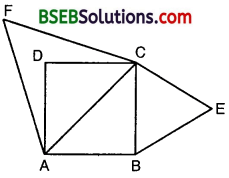Proof: ∆ BCE ~ ∆ ACF [All equilateral triangles are similar]Question 8.
ABC and BDE are two equilateral triangles such that D is the mid-point of BC. Ratio of the areas of triangles ABC and BDE is ____________.
(A) 2 : 1
(B) 1 : 2
(C) 4 : 1
(D) 1 : 4
Solution:
Since ∆ ABC and ∆ BDE are equilateral triangles, they are equiangular and hence ∆ ABC – ∆ BDE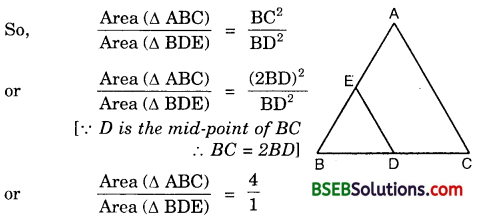∴ (C) is the correct answer.Question 9.
Sides of two similar triangles are in the ratio 4 : 9. Areas of these triangles are in the ratio
(A) 2 : 3
(B) 4 : 9
(C) 81 : 16
(D) 16 : 81
Solution:
Since the ratio of the areas of two similar triangles is equal to the ratio of the squares of any two corresponding sides, therefore
ratio of areas = (4)² : (9)² = 16 : 81
∴ (D) is the correct answer.